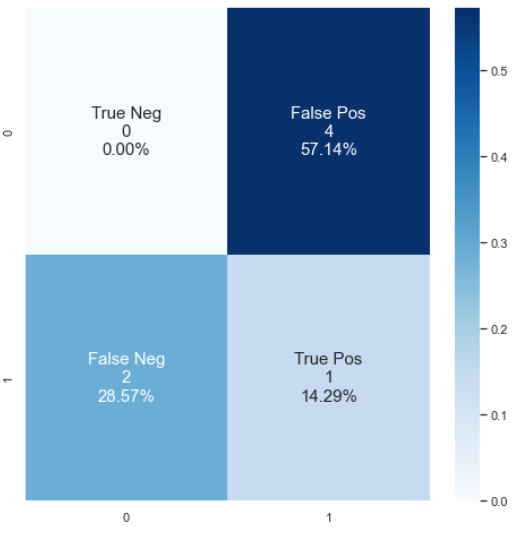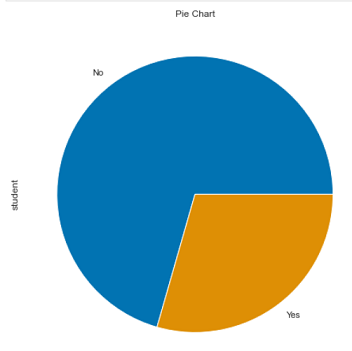# juspy Release 0.0.2b14

An EDA and modellling assist library

MIT
Install
``` pip install juspy==0.0.2b14 ```

# juspyjuspy is a library for making EDA and Modelling in Python quick and convinient. It's built on top of:

• pandas
• numpy
• seaborn
• and many such great libraries

Our reccomended path of getting started with `juspy`

• Open `Anaconda Prompt`
• In `Anaconda Prompt` type
`conda install -c conda-forge jupyterlab`
• and then
```pip install juspy
jupyterlab```

Incase if you're having any trouble regarding installation or dependencies, kindly make sure you're using updated versions

```conda update conda
conda update pip
conda update python```

And now try the above steps again

# Demo Notebooks:

1. To test library's proper installation and working:

```import juspy
print(juspy.__version__)

from juspy import greet
print(greet.namastey())```

Or, try greeting

```print(greet.hello())
print(greet.ni_hao())
print(greet.hola())
print(greet.bonjour())
print(greet.schatz())
print(greet.ahlan())
print(greet.privet())```

2. If they're producing some output, we're good to go
3. jpplot.confusion_matrix()

```import numpy as np
import seaborn as sns
from sklearn.metrics import confusion_matrix

y_true = [0, 1, 0, 1, 0, 1, 0]
y_pred = [1, 1, 1, 0, 1, 0, 1]
cf_matrix = confusion_matrix(y_true, y_pred)

from juspy import plot as jpplot
jpplot.confusion_matrix(cf_matrix)```4. jpplot.piechart()

```import pandas as pd`from juspy.linear_models import LinearRegression as lin_reg`